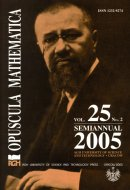Opuscula Math. 25, no. 2 (2005), 181-187

Opuscula Mathematica

# A note on geodesic and almost geodesic mappings of homogeneous Riemannian manifolds

Stanisław Formella

Abstract. Let $$M$$ be a differentiable manifold and denote by $$\nabla$$ and $$\tilde{\nabla}$$ two linear connections on $$M$$. $$\nabla$$ and $$\tilde{\nabla}$$ are said to be geodesically equivalent if and only if they have the same geodesics. A Riemannian manifold $$(M,g)$$ is a naturally reductive homogeneous manifold if and only if $$\nabla$$ and $$\tilde{\nabla}=\nabla-T$$ are geodesically equivalent, where $$T$$ is a homogeneous structure on $$(M,g)$$ ([Tricerri F., Vanhecke L., Homogeneous Structure on Riemannian Manifolds. London Math. Soc. Lecture Note Series, vol. 83, Cambridge Univ. Press 1983]). In the present paper we prove that if it is possible to map geodesically a homogeneous Riemannian manifold $$(M,g)$$ onto $$(M,\tilde{\nabla})$$, then the map is affine. If a naturally reductive manifold $$(M,g)$$ admits a nontrivial geodesic mapping onto a Riemannian manifold $$(\overline{M},\overline{g})$$ then both manifolds are of constant cutvature. We also give some results for almost geodesic mappings $$(M,g) \to (M,\tilde{\nabla})$$.

Keywords: homogeneous Riemannian manifold, geodesic, almost geodesic, geodesic mapping, almost geodesic mapping.

Mathematics Subject Classification: 53B30, 53C25.

Full text (pdf)

• Stanisław Formella
• Technical University, Institute of Mathematics, al. Piastów 48/49, 70-310 Szczecin, Poland